Anzeige

# Progressing towards efficiency: the role for labor tax progression in reforming social security

26. Mar 2023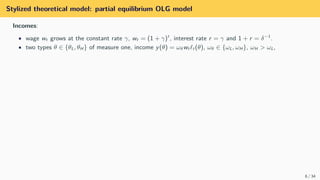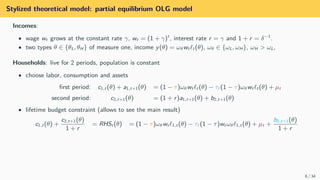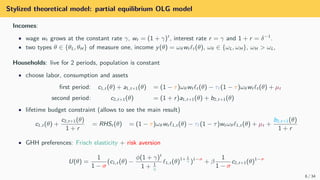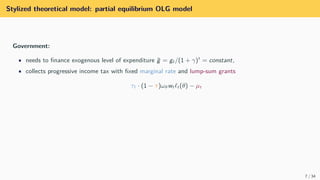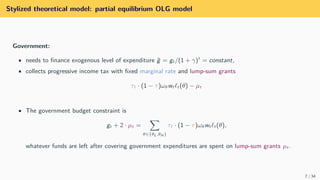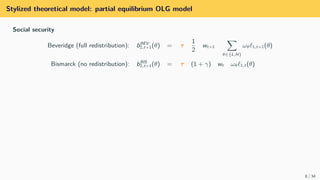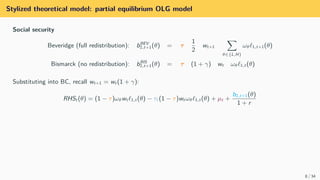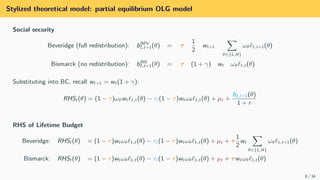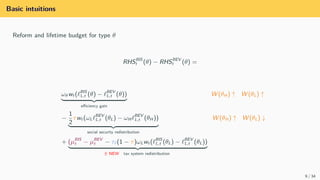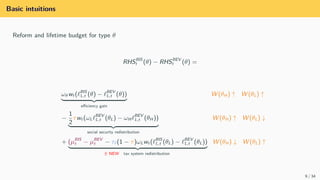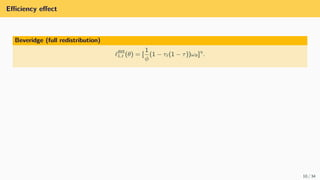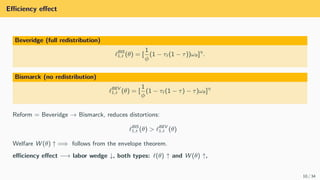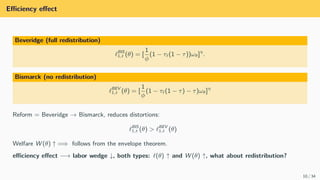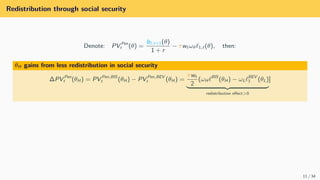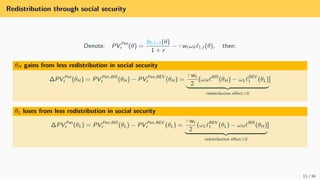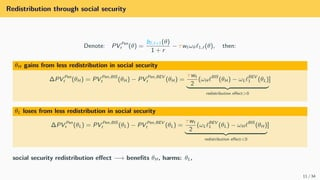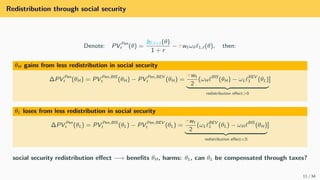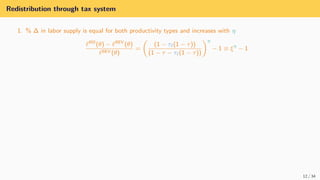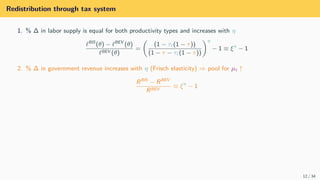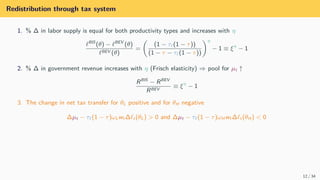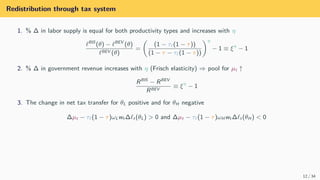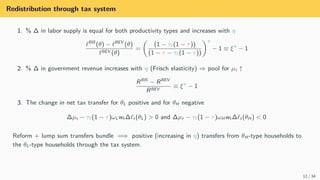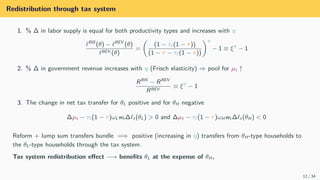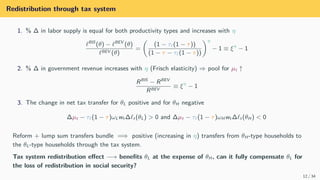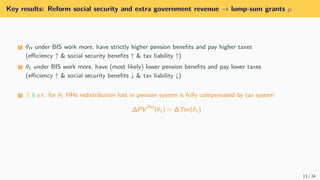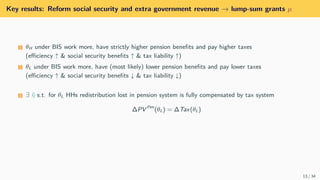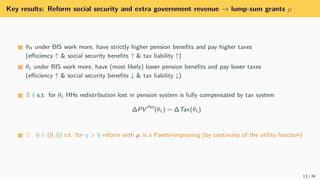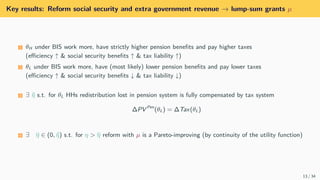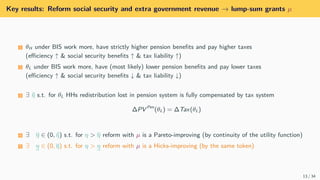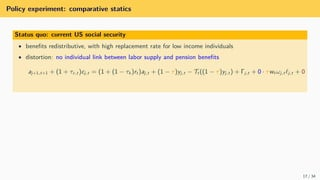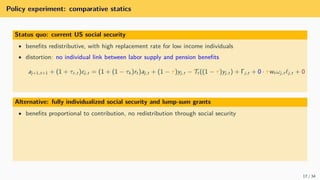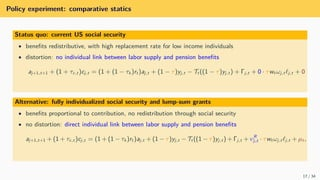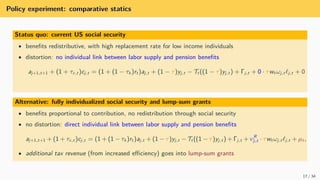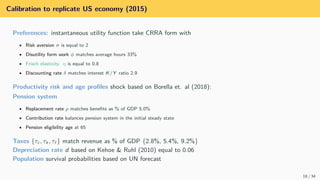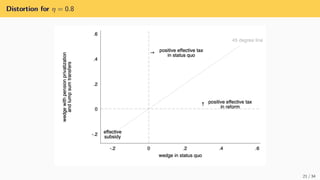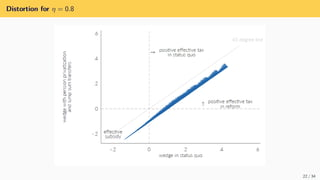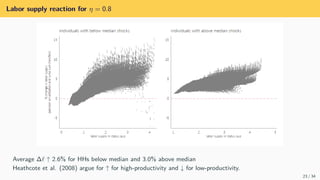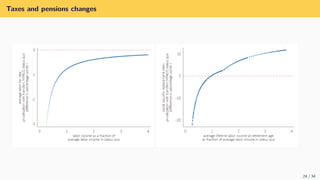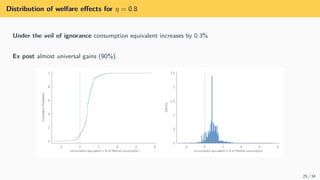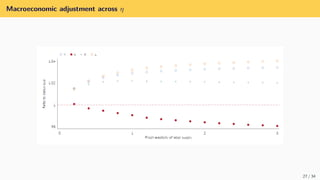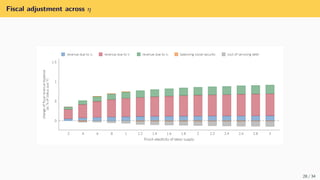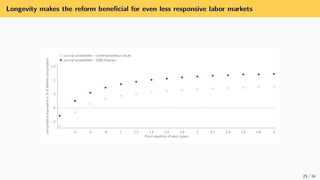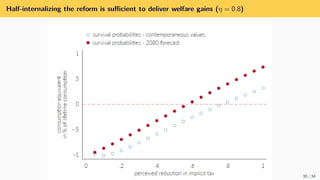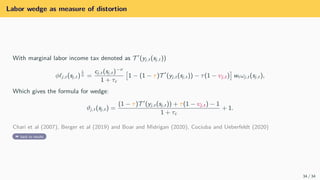1 von 77
Anzeige

### Progressing towards efficiency: the role for labor tax progression in reforming social security

1. Progressing into efficiency: the role for labor tax progression in privatizing social security Oliwia Komada (FAME|GRAPE) Krzysztof Makarski (FAME|GRAPE and Warsaw School of Economics) Joanna Tyrowicz (FAME|GRAPE, University of Regensburg, and IZA) EPCS, Hannover, 2023 1 / 34
2. Motivation 2 / 34
3. Motivation Social security is essentially about insurance: • old age (between cohorts) & mortality (annuitized) Benartzi et al. 2011, Bruce & Turnovsky 2013, Reichling & Smetters 2015, Caliendo et al. 2017 • low income (within cohort redistribution) Cooley & Soares 1996, Tabellini 2000 3 / 34
4. Motivation Social security is essentially about insurance: • old age (between cohorts) & mortality (annuitized) Benartzi et al. 2011, Bruce & Turnovsky 2013, Reichling & Smetters 2015, Caliendo et al. 2017 • low income (within cohort redistribution) Cooley & Soares 1996, Tabellini 2000 Prevailing consensus: • privatization of social security brings efficiency gains, • but reduces (within cohort) redistribution • this insurance loss reduces overall welfare effect of such reforms e.g. Nishiyama & Smetters (2007) 3 / 34
5. Motivation Social security is essentially about insurance: • old age (between cohorts) & mortality (annuitized) Benartzi et al. 2011, Bruce & Turnovsky 2013, Reichling & Smetters 2015, Caliendo et al. 2017 • low income (within cohort redistribution) Cooley & Soares 1996, Tabellini 2000 Prevailing consensus: • privatization of social security brings efficiency gains, • but reduces (within cohort) redistribution • this insurance loss reduces overall welfare effect of such reforms e.g. Nishiyama & Smetters (2007) Our approach: replace redistribution in social security with tax progression 3 / 34
6. Motivation Social security is essentially about insurance: • old age (between cohorts) & mortality (annuitized) Benartzi et al. 2011, Bruce & Turnovsky 2013, Reichling & Smetters 2015, Caliendo et al. 2017 • low income (within cohort redistribution) Cooley & Soares 1996, Tabellini 2000 Prevailing consensus: • privatization of social security brings efficiency gains, • but reduces (within cohort) redistribution • this insurance loss reduces overall welfare effect of such reforms e.g. Nishiyama & Smetters (2007) Our approach: replace redistribution in social security with tax progression Bottom line: shift insurance from retirement to working period → improve efficiency of social security → raise welfare. 3 / 34
8. Stylized theoretical model 5 / 34
9. Stylized theoretical model: partial equilibrium OLG model Incomes: • wage wt grows at the constant rate γ, wt = (1 + γ)t , interest rate r = γ and 1 + r = δ−1 . • two types θ ∈ {θL, θH } of measure one, income y(θ) = ωθwt ℓt (θ), ωθ ∈ {ωL, ωH }, ωH > ωL, 6 / 34
10. Stylized theoretical model: partial equilibrium OLG model Incomes: • wage wt grows at the constant rate γ, wt = (1 + γ)t , interest rate r = γ and 1 + r = δ−1 . • two types θ ∈ {θL, θH } of measure one, income y(θ) = ωθwt ℓt (θ), ωθ ∈ {ωL, ωH }, ωH > ωL, Households: live for 2 periods, population is constant • choose labor, consumption and assets first period: c1,t (θ) + a1,t+1(θ) = (1 − τ)ωθwt ℓt (θ) − τℓ(1 − τ)ωθwt ℓt (θ) + µt second period: c2,t+1(θ) = (1 + r)a1,t+1(θ) + b2,t+1(θ) • lifetime budget constraint (allows to see the main result) c1,t (θ) + c2,t+1(θ) 1 + r = RHSt (θ) = (1 − τ)ωθwt ℓ1,t (θ) − τℓ(1 − τ)wt ωθℓ1,t (θ) + µt + b2,t+1(θ) 1 + r 6 / 34
11. Stylized theoretical model: partial equilibrium OLG model Incomes: • wage wt grows at the constant rate γ, wt = (1 + γ)t , interest rate r = γ and 1 + r = δ−1 . • two types θ ∈ {θL, θH } of measure one, income y(θ) = ωθwt ℓt (θ), ωθ ∈ {ωL, ωH }, ωH > ωL, Households: live for 2 periods, population is constant • choose labor, consumption and assets first period: c1,t (θ) + a1,t+1(θ) = (1 − τ)ωθwt ℓt (θ) − τℓ(1 − τ)ωθwt ℓt (θ) + µt second period: c2,t+1(θ) = (1 + r)a1,t+1(θ) + b2,t+1(θ) • lifetime budget constraint (allows to see the main result) c1,t (θ) + c2,t+1(θ) 1 + r = RHSt (θ) = (1 − τ)ωθwt ℓ1,t (θ) − τℓ(1 − τ)wt ωθℓ1,t (θ) + µt + b2,t+1(θ) 1 + r • GHH preferences: Frisch elasticity + risk aversion U(θ) = 1 1 − σ (c1,t (θ) − ϕ(1 + γ)t 1 + 1 η ℓ1,t (θ) 1+ 1 η )1−σ + β 1 1 − σ c2,t+1(θ)1−σ 6 / 34
12. Stylized theoretical model: partial equilibrium OLG model Government: • needs to finance exogenous level of expenditure g̃ = gt /(1 + γ)t = constant, • collects progressive income tax with fixed marginal rate and lump-sum grants τℓ · (1 − τ)ωθwt ℓt (θ) − µt 7 / 34
13. Stylized theoretical model: partial equilibrium OLG model Government: • needs to finance exogenous level of expenditure g̃ = gt /(1 + γ)t = constant, • collects progressive income tax with fixed marginal rate and lump-sum grants τℓ · (1 − τ)ωθwt ℓt (θ) − µt • The government budget constraint is gt + 2 · µt = X θ∈{θL,θH } τℓ · (1 − τ)ωθwt ℓt (θ), whatever funds are left after covering government expenditures are spent on lump-sum grants µt . 7 / 34
14. Stylized theoretical model: partial equilibrium OLG model Social security Beveridge (full redistribution): bBEV 2,t+1(θ) = τ 1 2 wt+1 X θ∈{L,H} ωθℓ1,t+1(θ) Bismarck (no redistribution): bBIS 2,t+1(θ) = τ (1 + γ) wt ωθℓ1,t (θ) 8 / 34
15. Stylized theoretical model: partial equilibrium OLG model Social security Beveridge (full redistribution): bBEV 2,t+1(θ) = τ 1 2 wt+1 X θ∈{L,H} ωθℓ1,t+1(θ) Bismarck (no redistribution): bBIS 2,t+1(θ) = τ (1 + γ) wt ωθℓ1,t (θ) Substituting into BC, recall wt+1 = wt (1 + γ): RHSt (θ) = (1 − τ)ωθwt ℓ1,t (θ) − τℓ(1 − τ)wt ωθℓ1,t (θ) + µt + b2,t+1(θ) 1 + r 8 / 34
16. Stylized theoretical model: partial equilibrium OLG model Social security Beveridge (full redistribution): bBEV 2,t+1(θ) = τ 1 2 wt+1 X θ∈{L,H} ωθℓ1,t+1(θ) Bismarck (no redistribution): bBIS 2,t+1(θ) = τ (1 + γ) wt ωθℓ1,t (θ) Substituting into BC, recall wt+1 = wt (1 + γ): RHSt (θ) = (1 − τ)ωθwt ℓ1,t (θ) − τℓ(1 − τ)wt ωθℓ1,t (θ) + µt + b2,t+1(θ) 1 + r RHS of Lifetime Budget Beveridge: RHSt (θ) = (1 − τ)wt ωθℓ1,t (θ) − τℓ(1 − τ)wt ωθℓ1,t (θ) + µt + τ 1 2 wt X θ∈{L,H} ωθℓ1,t+1(θ) Bismarck: RHSt (θ) = (1 − τ)wt ωθℓ1,t (θ) − τℓ(1 − τ)wt ωθℓ1,t (θ) + µt + τwt ωθℓ1,t (θ) 8 / 34
17. Basic intuitions Reform and lifetime budget for type θ RHSBIS t (θ) − RHSBEV t (θ) = 9 / 34
18. Basic intuitions Reform and lifetime budget for type θ RHSBIS t (θ) − RHSBEV t (θ) = ωθwt (ℓBIS 1,t (θ) − ℓBEV 1,t (θ)) | {z } efficiency gain 9 / 34
19. Basic intuitions Reform and lifetime budget for type θ RHSBIS t (θ) − RHSBEV t (θ) = ωθwt (ℓBIS 1,t (θ) − ℓBEV 1,t (θ)) | {z } efficiency gain W (θH ) ↑ W (θL) ↑ 9 / 34
20. Basic intuitions Reform and lifetime budget for type θ RHSBIS t (θ) − RHSBEV t (θ) = ωθwt (ℓBIS 1,t (θ) − ℓBEV 1,t (θ)) | {z } efficiency gain W (θH ) ↑ W (θL) ↑ − 1 2 τwt (ωLℓBEV 1,t (θL) − ωH ℓBEV 1,t (θH )) | {z } social security redistribution 9 / 34
21. Basic intuitions Reform and lifetime budget for type θ RHSBIS t (θ) − RHSBEV t (θ) = ωθwt (ℓBIS 1,t (θ) − ℓBEV 1,t (θ)) | {z } efficiency gain W (θH ) ↑ W (θL) ↑ − 1 2 τwt (ωLℓBEV 1,t (θL) − ωH ℓBEV 1,t (θH )) | {z } social security redistribution W (θH ) ↑ W (θL) ↓ 9 / 34
22. Basic intuitions Reform and lifetime budget for type θ RHSBIS t (θ) − RHSBEV t (θ) = ωθwt (ℓBIS 1,t (θ) − ℓBEV 1,t (θ)) | {z } efficiency gain W (θH ) ↑ W (θL) ↑ − 1 2 τwt (ωLℓBEV 1,t (θL) − ωH ℓBEV 1,t (θH )) | {z } social security redistribution W (θH ) ↑ W (θL) ↓ + (µBIS t − µBEV t − τℓ(1 − τ)ωLwt (ℓBIS 1,t (θL) − ℓBEV 1,t (θL)) | {z } ⇑ NEW tax system redistribution 9 / 34
23. Basic intuitions Reform and lifetime budget for type θ RHSBIS t (θ) − RHSBEV t (θ) = ωθwt (ℓBIS 1,t (θ) − ℓBEV 1,t (θ)) | {z } efficiency gain W (θH ) ↑ W (θL) ↑ − 1 2 τwt (ωLℓBEV 1,t (θL) − ωH ℓBEV 1,t (θH )) | {z } social security redistribution W (θH ) ↑ W (θL) ↓ + (µBIS t − µBEV t − τℓ(1 − τ)ωLwt (ℓBIS 1,t (θL) − ℓBEV 1,t (θL)) | {z } ⇑ NEW tax system redistribution W (θH ) ↓ W (θL) ↑ 9 / 34
24. Efficiency effect Beveridge (full redistribution) ℓBIS 1,t (θ) = [ 1 ϕ (1 − τℓ(1 − τ))ωθ]η . 10 / 34
25. Efficiency effect Beveridge (full redistribution) ℓBIS 1,t (θ) = [ 1 ϕ (1 − τℓ(1 − τ))ωθ]η . Bismarck (no redistribution) ℓBEV 1,t (θ) = [ 1 ϕ (1 − τℓ(1 − τ) − τ)ωθ]η Reform = Beveridge → Bismarck, reduces distortions: ℓBIS 1,t (θ) > ℓBEV 1,t (θ) Welfare W (θ) ↑ =⇒ follows from the envelope theorem. efficiency effect −→ labor wedge ↓, both types: ℓ(θ) ↑ and W (θ) ↑, 10 / 34
26. Efficiency effect Beveridge (full redistribution) ℓBIS 1,t (θ) = [ 1 ϕ (1 − τℓ(1 − τ))ωθ]η . Bismarck (no redistribution) ℓBEV 1,t (θ) = [ 1 ϕ (1 − τℓ(1 − τ) − τ)ωθ]η Reform = Beveridge → Bismarck, reduces distortions: ℓBIS 1,t (θ) > ℓBEV 1,t (θ) Welfare W (θ) ↑ =⇒ follows from the envelope theorem. efficiency effect −→ labor wedge ↓, both types: ℓ(θ) ↑ and W (θ) ↑, what about redistribution? 10 / 34
27. Redistribution through social security Denote: PV Pen t (θ) = b2,t+1(θ) 1 + r − τwt ωθℓ1,t (θ), then: 11 / 34
28. Redistribution through social security Denote: PV Pen t (θ) = b2,t+1(θ) 1 + r − τwt ωθℓ1,t (θ), then: θH gains from less redistribution in social security ∆PV Pen t (θH ) = PV Pen,BIS t (θH ) − PV Pen,BEV t (θH ) = τwt 2 (ωH ℓBIS (θH ) − ωLℓBEV 1 (θL) | {z } redistribution effect>0 ] 11 / 34
29. Redistribution through social security Denote: PV Pen t (θ) = b2,t+1(θ) 1 + r − τwt ωθℓ1,t (θ), then: θH gains from less redistribution in social security ∆PV Pen t (θH ) = PV Pen,BIS t (θH ) − PV Pen,BEV t (θH ) = τwt 2 (ωH ℓBIS (θH ) − ωLℓBEV 1 (θL) | {z } redistribution effect>0 ] θL loses from less redistribution in social security ∆PV Pen t (θL) = PV Pen,BIS t (θL) − PV Pen,BEV t (θL) = τwt 2 (ωLℓBEV 1 (θL) − ωH ℓBIS (θH ) | {z } redistribution effect<0 ] 11 / 34
30. Redistribution through social security Denote: PV Pen t (θ) = b2,t+1(θ) 1 + r − τwt ωθℓ1,t (θ), then: θH gains from less redistribution in social security ∆PV Pen t (θH ) = PV Pen,BIS t (θH ) − PV Pen,BEV t (θH ) = τwt 2 (ωH ℓBIS (θH ) − ωLℓBEV 1 (θL) | {z } redistribution effect>0 ] θL loses from less redistribution in social security ∆PV Pen t (θL) = PV Pen,BIS t (θL) − PV Pen,BEV t (θL) = τwt 2 (ωLℓBEV 1 (θL) − ωH ℓBIS (θH ) | {z } redistribution effect<0 ] social security redistribution effect −→ benefits θH , harms: θL, 11 / 34
31. Redistribution through social security Denote: PV Pen t (θ) = b2,t+1(θ) 1 + r − τwt ωθℓ1,t (θ), then: θH gains from less redistribution in social security ∆PV Pen t (θH ) = PV Pen,BIS t (θH ) − PV Pen,BEV t (θH ) = τwt 2 (ωH ℓBIS (θH ) − ωLℓBEV 1 (θL) | {z } redistribution effect>0 ] θL loses from less redistribution in social security ∆PV Pen t (θL) = PV Pen,BIS t (θL) − PV Pen,BEV t (θL) = τwt 2 (ωLℓBEV 1 (θL) − ωH ℓBIS (θH ) | {z } redistribution effect<0 ] social security redistribution effect −→ benefits θH , harms: θL, can θL be compensated through taxes? 11 / 34
32. Redistribution through tax system 1. % ∆ in labor supply is equal for both productivity types and increases with η ℓBIS (θ) − ℓBEV (θ) ℓBEV (θ) = (1 − τℓ(1 − τ)) (1 − τ − τℓ(1 − τ)) η − 1 ≡ ξη − 1 12 / 34
33. Redistribution through tax system 1. % ∆ in labor supply is equal for both productivity types and increases with η ℓBIS (θ) − ℓBEV (θ) ℓBEV (θ) = (1 − τℓ(1 − τ)) (1 − τ − τℓ(1 − τ)) η − 1 ≡ ξη − 1 2. % ∆ in government revenue increases with η (Frisch elasticity) ⇒ pool for µt ↑ RBIS − RBEV RBEV ≡ ξη − 1 12 / 34
34. Redistribution through tax system 1. % ∆ in labor supply is equal for both productivity types and increases with η ℓBIS (θ) − ℓBEV (θ) ℓBEV (θ) = (1 − τℓ(1 − τ)) (1 − τ − τℓ(1 − τ)) η − 1 ≡ ξη − 1 2. % ∆ in government revenue increases with η (Frisch elasticity) ⇒ pool for µt ↑ RBIS − RBEV RBEV ≡ ξη − 1 3. The change in net tax transfer for θL positive and for θH negative ∆µt − τℓ(1 − τ)ωLwt ∆ℓt (θL) 0 and ∆µt − τℓ(1 − τ)ωH wt ∆ℓt (θH ) 0 12 / 34
35. Redistribution through tax system 1. % ∆ in labor supply is equal for both productivity types and increases with η ℓBIS (θ) − ℓBEV (θ) ℓBEV (θ) = (1 − τℓ(1 − τ)) (1 − τ − τℓ(1 − τ)) η − 1 ≡ ξη − 1 2. % ∆ in government revenue increases with η (Frisch elasticity) ⇒ pool for µt ↑ RBIS − RBEV RBEV ≡ ξη − 1 3. The change in net tax transfer for θL positive and for θH negative ∆µt − τℓ(1 − τ)ωLwt ∆ℓt (θL) 0 and ∆µt − τℓ(1 − τ)ωH wt ∆ℓt (θH ) 0 12 / 34
36. Redistribution through tax system 1. % ∆ in labor supply is equal for both productivity types and increases with η ℓBIS (θ) − ℓBEV (θ) ℓBEV (θ) = (1 − τℓ(1 − τ)) (1 − τ − τℓ(1 − τ)) η − 1 ≡ ξη − 1 2. % ∆ in government revenue increases with η (Frisch elasticity) ⇒ pool for µt ↑ RBIS − RBEV RBEV ≡ ξη − 1 3. The change in net tax transfer for θL positive and for θH negative ∆µt − τℓ(1 − τ)ωLwt ∆ℓt (θL) 0 and ∆µt − τℓ(1 − τ)ωH wt ∆ℓt (θH ) 0 Reform + lump sum transfers bundle =⇒ positive (increasing in η) transfers from θH -type households to the θL-type households through the tax system. 12 / 34
37. Redistribution through tax system 1. % ∆ in labor supply is equal for both productivity types and increases with η ℓBIS (θ) − ℓBEV (θ) ℓBEV (θ) = (1 − τℓ(1 − τ)) (1 − τ − τℓ(1 − τ)) η − 1 ≡ ξη − 1 2. % ∆ in government revenue increases with η (Frisch elasticity) ⇒ pool for µt ↑ RBIS − RBEV RBEV ≡ ξη − 1 3. The change in net tax transfer for θL positive and for θH negative ∆µt − τℓ(1 − τ)ωLwt ∆ℓt (θL) 0 and ∆µt − τℓ(1 − τ)ωH wt ∆ℓt (θH ) 0 Reform + lump sum transfers bundle =⇒ positive (increasing in η) transfers from θH -type households to the θL-type households through the tax system. Tax system redistribution effect −→ benefits θL at the expense of θH , 12 / 34
38. Redistribution through tax system 1. % ∆ in labor supply is equal for both productivity types and increases with η ℓBIS (θ) − ℓBEV (θ) ℓBEV (θ) = (1 − τℓ(1 − τ)) (1 − τ − τℓ(1 − τ)) η − 1 ≡ ξη − 1 2. % ∆ in government revenue increases with η (Frisch elasticity) ⇒ pool for µt ↑ RBIS − RBEV RBEV ≡ ξη − 1 3. The change in net tax transfer for θL positive and for θH negative ∆µt − τℓ(1 − τ)ωLwt ∆ℓt (θL) 0 and ∆µt − τℓ(1 − τ)ωH wt ∆ℓt (θH ) 0 Reform + lump sum transfers bundle =⇒ positive (increasing in η) transfers from θH -type households to the θL-type households through the tax system. Tax system redistribution effect −→ benefits θL at the expense of θH , can it fully compensate θL for the loss of redistribution in social security? 12 / 34
39. Key results: Reform social security and extra government revenue → lump-sum grants µ 1 θH under BIS work more, have strictly higher pension benefits and pay higher taxes (efficiency ↑ social security benefits ↑ tax liability ↑) 13 / 34
40. Key results: Reform social security and extra government revenue → lump-sum grants µ 1 θH under BIS work more, have strictly higher pension benefits and pay higher taxes (efficiency ↑ social security benefits ↑ tax liability ↑) 2 θL under BIS work more, have (most likely) lower pension benefits and pay lower taxes (efficiency ↑ social security benefits ↓ tax liability ↓) 13 / 34
41. Key results: Reform social security and extra government revenue → lump-sum grants µ 1 θH under BIS work more, have strictly higher pension benefits and pay higher taxes (efficiency ↑ social security benefits ↑ tax liability ↑) 2 θL under BIS work more, have (most likely) lower pension benefits and pay lower taxes (efficiency ↑ social security benefits ↓ tax liability ↓) 13 / 34
42. Key results: Reform social security and extra government revenue → lump-sum grants µ 1 θH under BIS work more, have strictly higher pension benefits and pay higher taxes (efficiency ↑ social security benefits ↑ tax liability ↑) 2 θL under BIS work more, have (most likely) lower pension benefits and pay lower taxes (efficiency ↑ social security benefits ↓ tax liability ↓) 3 ∃ η̃ s.t. for θL HHs redistribution lost in pension system is fully compensated by tax system ∆PV Pen (θL) = ∆Tax(θL) 13 / 34
43. Key results: Reform social security and extra government revenue → lump-sum grants µ 1 θH under BIS work more, have strictly higher pension benefits and pay higher taxes (efficiency ↑ social security benefits ↑ tax liability ↑) 2 θL under BIS work more, have (most likely) lower pension benefits and pay lower taxes (efficiency ↑ social security benefits ↓ tax liability ↓) 3 ∃ η̃ s.t. for θL HHs redistribution lost in pension system is fully compensated by tax system ∆PV Pen (θL) = ∆Tax(θL) 13 / 34
44. Key results: Reform social security and extra government revenue → lump-sum grants µ 1 θH under BIS work more, have strictly higher pension benefits and pay higher taxes (efficiency ↑ social security benefits ↑ tax liability ↑) 2 θL under BIS work more, have (most likely) lower pension benefits and pay lower taxes (efficiency ↑ social security benefits ↓ tax liability ↓) 3 ∃ η̃ s.t. for θL HHs redistribution lost in pension system is fully compensated by tax system ∆PV Pen (θL) = ∆Tax(θL) 4 ∃ η ∈ (0, η̃) s.t. for η η reform with µ is a Pareto-improving (by continuity of the utility function) 13 / 34
45. Key results: Reform social security and extra government revenue → lump-sum grants µ 1 θH under BIS work more, have strictly higher pension benefits and pay higher taxes (efficiency ↑ social security benefits ↑ tax liability ↑) 2 θL under BIS work more, have (most likely) lower pension benefits and pay lower taxes (efficiency ↑ social security benefits ↓ tax liability ↓) 3 ∃ η̃ s.t. for θL HHs redistribution lost in pension system is fully compensated by tax system ∆PV Pen (θL) = ∆Tax(θL) 4 ∃ η ∈ (0, η̃) s.t. for η η reform with µ is a Pareto-improving (by continuity of the utility function) 13 / 34
46. Key results: Reform social security and extra government revenue → lump-sum grants µ 1 θH under BIS work more, have strictly higher pension benefits and pay higher taxes (efficiency ↑ social security benefits ↑ tax liability ↑) 2 θL under BIS work more, have (most likely) lower pension benefits and pay lower taxes (efficiency ↑ social security benefits ↓ tax liability ↓) 3 ∃ η̃ s.t. for θL HHs redistribution lost in pension system is fully compensated by tax system ∆PV Pen (θL) = ∆Tax(θL) 4 ∃ η ∈ (0, η̃) s.t. for η η reform with µ is a Pareto-improving (by continuity of the utility function) 5 ∃ η ∈ (0, η) s.t. for η η reform with µ is a Hicks-improving (by the same token) 13 / 34
47. Quantitative model 14 / 34
48. Quantitative model Consumers • uncertain lifetimes: live for 16 periods, with survival πj 1 • ex ante heterogeneous productivity + uninsurable productivity risk • consume, work and save based on CRRA instantaneous utility function 1 1−σ c1−σ − ϕ 1+1/η ℓ1+1/η • pay taxes (progressive on labor, linear on consumption and capital gains) • contribute to social security, face natural borrowing constraint 15 / 34
49. Quantitative model Consumers • uncertain lifetimes: live for 16 periods, with survival πj 1 • ex ante heterogeneous productivity + uninsurable productivity risk • consume, work and save based on CRRA instantaneous utility function 1 1−σ c1−σ − ϕ 1+1/η ℓ1+1/η • pay taxes (progressive on labor, linear on consumption and capital gains) • contribute to social security, face natural borrowing constraint Firms and markets • Cobb-Douglas production function, capital depreciates at rate d • no annuity, financial markets with (risk free) interest rate 15 / 34
50. Quantitative model Government • Finances government spending Gt , constant between scenarios, • Balances pension system: subsidyt • Services debt: rt Dt , • Collects taxes on capital, consumption, labor, and covers lump-sum grant (progressive labor tax given by Benabou form) Gt + subsidyt + rt Dt + Mt = τk,t rt At + τc,t Ct + Taxℓ,t + ∆Dt where ∆Dt = Dt − Dt−1 16 / 34
51. Policy experiment: comparative statics Status quo: current US social security • benefits redistributive, with high replacement rate for low income individuals 17 / 34
52. Policy experiment: comparative statics Status quo: current US social security • benefits redistributive, with high replacement rate for low income individuals • distortion: no individual link between labor supply and pension benefits aj+1,t+1 + (1 + τc,t )cj,t = (1 + (1 − τk )rt )aj,t + (1 − τ)yj,t − Tt ((1 − τ)yj,t ) + Γj,t + 0 · τwt ωj,t ℓj,t + 0 17 / 34
53. Policy experiment: comparative statics Status quo: current US social security • benefits redistributive, with high replacement rate for low income individuals • distortion: no individual link between labor supply and pension benefits aj+1,t+1 + (1 + τc,t )cj,t = (1 + (1 − τk )rt )aj,t + (1 − τ)yj,t − Tt ((1 − τ)yj,t ) + Γj,t + 0 · τwt ωj,t ℓj,t + 0 17 / 34
54. Policy experiment: comparative statics Status quo: current US social security • benefits redistributive, with high replacement rate for low income individuals • distortion: no individual link between labor supply and pension benefits aj+1,t+1 + (1 + τc,t )cj,t = (1 + (1 − τk )rt )aj,t + (1 − τ)yj,t − Tt ((1 − τ)yj,t ) + Γj,t + 0 · τwt ωj,t ℓj,t + 0 Alternative: fully individualized social security and lump-sum grants • benefits proportional to contribution, no redistribution through social security 17 / 34
55. Policy experiment: comparative statics Status quo: current US social security • benefits redistributive, with high replacement rate for low income individuals • distortion: no individual link between labor supply and pension benefits aj+1,t+1 + (1 + τc,t )cj,t = (1 + (1 − τk )rt )aj,t + (1 − τ)yj,t − Tt ((1 − τ)yj,t ) + Γj,t + 0 · τwt ωj,t ℓj,t + 0 Alternative: fully individualized social security and lump-sum grants • benefits proportional to contribution, no redistribution through social security • no distortion: direct individual link between labor supply and pension benefits aj+1,t+1 + (1 + τc,t )cj,t = (1 + (1 − τk )rt )aj,t + (1 − τ)yj,t − Tt ((1 − τ)yj,t ) + Γj,t + υR j,t · τwt ωj,t ℓj,t + µt , 17 / 34
56. Policy experiment: comparative statics Status quo: current US social security • benefits redistributive, with high replacement rate for low income individuals • distortion: no individual link between labor supply and pension benefits aj+1,t+1 + (1 + τc,t )cj,t = (1 + (1 − τk )rt )aj,t + (1 − τ)yj,t − Tt ((1 − τ)yj,t ) + Γj,t + 0 · τwt ωj,t ℓj,t + 0 Alternative: fully individualized social security and lump-sum grants • benefits proportional to contribution, no redistribution through social security • no distortion: direct individual link between labor supply and pension benefits aj+1,t+1 + (1 + τc,t )cj,t = (1 + (1 − τk )rt )aj,t + (1 − τ)yj,t − Tt ((1 − τ)yj,t ) + Γj,t + υR j,t · τwt ωj,t ℓj,t + µt , • additional tax revenue (from increased efficiency) goes into lump-sum grants 17 / 34
57. Calibration to replicate US economy (2015) Preferences: instantaneous utility function take CRRA form with • Risk aversion σ is equal to 2 • Disutility form work ϕ matches average hours 33% • Frisch elasticity η is equal to 0.8 • Discounting rate δ matches interest K/Y ratio 2.9 Productivity risk and age profiles shock based on Borella et. al (2018): Pension system • Replacement rate ρ matches benefits as % of GDP 5.0% • Contribution rate balances pension system in the initial steady state • Pension eligibility age at 65 Taxes {τc , τk , τℓ} match revenue as % of GDP {2.8%, 5.4%, 9.2%} Depreciation rate d based on Kehoe Ruhl (2010) equal to 0.06 Population survival probabilities based on UN forecast 18 / 34
58. Results 19 / 34
59. Distortion for η = 0.8 labor wedge formula 20 / 34
60. Distortion for η = 0.8 21 / 34
61. Distortion for η = 0.8 22 / 34
62. Labor supply reaction for η = 0.8 Average ∆ℓ ↑ 2.6% for HHs below median and 3.0% above median Heathcote et al. (2008) argue for ↑ for high-productivity and ↓ for low-productivity. 23 / 34
63. Taxes and pensions changes 24 / 34
64. Distribution of welfare effects for η = 0.8 Under the veil of ignorance consumption equivalent increases by 0.3% 25 / 34
65. Distribution of welfare effects for η = 0.8 Under the veil of ignorance consumption equivalent increases by 0.3% Ex post almost universal gains (90%). 25 / 34
66. Welfare effect across η 26 / 34
67. Macroeconomic adjustment across η 27 / 34
68. Fiscal adjustment across η 28 / 34
69. Longevity makes the reform beneficial for even less responsive labor markets 29 / 34
70. Half-internalizing the reform is sufficient to deliver welfare gains (η = 0.8) 30 / 34
71. Conclusions 31 / 34
72. Conclusions 1. Progression in the tax system can effectively substitute for progression in social security ... 32 / 34
73. Conclusions 1. Progression in the tax system can effectively substitute for progression in social security ... 2. ... generating welfare gains 32 / 34
74. Conclusions 1. Progression in the tax system can effectively substitute for progression in social security ... 2. ... generating welfare gains 3. With rising longevity, the potential welfare gains are higher. 32 / 34
75. Conclusions 1. Progression in the tax system can effectively substitute for progression in social security ... 2. ... generating welfare gains 3. With rising longevity, the potential welfare gains are higher. 4. Important role for response of labor to the features of the pension system 32 / 34
76. Questions or suggestions? Thank you! w: grape.org.pl t: grape org f: grape.org e: j.tyrowicz@grape.org.pl 33 / 34
77. Labor wedge as measure of distortion With marginal labor income tax denoted as T ′ (yj,t (sj,t )) ϕℓj,t (sj,t ) 1 η = cj,t (sj,t )−σ 1 + τc 1 − (1 − τ)T ′ (yj,t (sj,t )) − τ(1 − υj,t ) wt ωj,t (sj,t ), Which gives the formula for wedge: ϑj,t (sj,t ) = (1 − τ)T ′ (yj,t (sj,t )) + τ(1 − υj,t ) − 1 1 + τc + 1. Chari et al (2007), Berger et al (2019) and Boar and Midrigan (2020), Cociuba and Ueberfeldt (2020) back to results 34 / 34
Anzeige